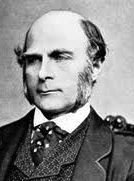# Galton-Watson-Bienayme processes

Sir Frances Galton was a cousin of Charles Darwin and an eminent scientist. He is responsible for the idea of regression to the mean'' which now gives the name for the statistical methods of regression analysis. Galton worked with Watson to describe the process of extinction of family names -- working in a world where sons inherited familiy names from fathers and women changed their names on marriage. The model then supposes that generation 0 is a single man. In generation 1 there are Z_1 sons of the first man generated from some offspring'' distribution $p(k)=P(Z=k)$. Independently of each other each of these sons has $Z_{2,i}$ sons of his own for $i=1,...,Z_1$, again according to the distribution $p$. We actually observe only $Z_2=\sum_i Z_{2,i}$. In the third generation second generation male number $i$ generates $Z_{3,i}$ offspring independently of all others and so on.

In other words generation number $n+1$ has total size $Z_{n+1}$ which is a sum of $Z_n$ independent and identically distributed numbers of sons $Z_{n+1,i}$; each of these has distribution $p$. I have worked on inference in these processes with Peter Guttorp . Peter has written a great book about inference problems in these (and more general) processes: Statistical inference for branching processes, Wiley, 1991.

My own role with Peter was in investigating inference for $p$. The results we have here are mostly negative, showing the non-existence of consistent estimates of parameters of the offspring distribution other than the mean and variance. We have looked at the maximum likelihood estimate of the variance of the offspring distribution. Though this offspring distribution is not consistently estimable its mean and variance are. It is known that mle of the mean is consistent and we think we can prove that the mle of the variance is also consistent. Our proof relies on a uniform approximation of the likelihood based on a uniform version of the local central limit theorem.

Lockhart, R. A. (1982). On the non-existence of consistent estimates in Galton-Watson processes. J. Appl. Prob., 19 842--846.
Draft paper# Sparsely sampled random walks

I like to work on large sample theory with what I think of as clean, simple conditions. Peter Guttorp and I did this sort of thing in work on inference in irregularly sampled random walks. That work was motivated by a paper I wrote applying the local central limit theorem to prove that on an explosive trajectory of a Bienaym\'e-Galton-Watson only the mean, variance and perhaps one other arithmetic parameter admit consistent estimates.

Guttorp, P. and Lockhart, R.A. (1989). Estimation in sparsely sampled random walks. Stoch. Proc. Their Appl., 31 315--320.